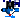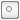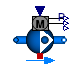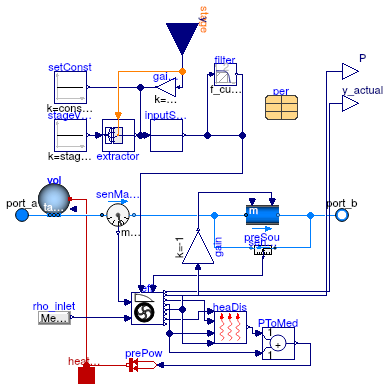## Buildings.Fluid.Movers

Package with fan and pump models

### Information

This package contains component models for fans and pumps.

Extends from Modelica.Icons.Package (Icon for standard packages).

### Package Content

Name DescriptionUsersGuide User's GuideFlowControlled_dp Fan or pump with ideally controlled head dp as input signalFlowControlled_m_flow Fan or pump with ideally controlled mass flow rate as input signalSpeedControlled_Nrpm Fan or pump with ideally controlled speed Nrpm as input signalSpeedControlled_y Fan or pump with ideally controlled normalized speed y as input signalData Package containing data for real pumps/fansExamples Collection of models that illustrate model use and test modelsValidation Collection of validation modelsBaseClasses Package with base classes for Buildings.Fluid.Movers

##Buildings.Fluid.Movers.FlowControlled_dp

Fan or pump with ideally controlled head dp as input signal### Information

This model describes a fan or pump with prescribed head. The input connector provides the difference between outlet minus inlet pressure. The efficiency of the device is computed based on the efficiency and pressure curves that are defined in record `per`, which is of type Buildings.Fluid.Movers.SpeedControlled_Nrpm.

#### Main equations

See the User's Guide.

#### Typical use and important parameters

If `use_inputFilter=true`, then the parameter `dp_nominal` is used to normalize the filter. This is used to improve the numerics of the transient response. The actual pressure raise of the mover at steady-state is independent of the value of `dp_nominal`. It is recommended to set `dp_nominal` to approximately the pressure raise that the mover has during full speed.

#### Options

Parameter `prescribeSystemPressure` can be used to control the mover such that the pressure difference set point is obtained across two points in the system, instead of across the fan. This allows an efficient implementation of static pressure reset controllers. A measurement of the pressure difference between the two points in system then needs to be connected to `RealInput dpMea`. This functionality is demonstrated in Buildings.Fluid.Movers.Validation.FlowControlled_dpSystem.

Extends from Buildings.Fluid.Movers.BaseClasses.PartialFlowMachine (Partial model to interface fan or pump models with the medium).

### Parameters

TypeNameDefaultDescription
replaceable package MediumPartialMediumMedium in the component
Genericperredeclare parameter Building...Record with performance data
BooleancomputePowerUsingSimilarityLawsper.havePressureCurve= true, compute power exactly, using similarity laws. Otherwise approximate.
BooleanaddPowerToMediumtrueSet to false to avoid any power (=heat and flow work) being added to medium (may give simpler equations)
BooleannominalValuesDefineDefaultPressureCurvefalseSet to true to avoid warning if m_flow_nominal and dp_nominal are used to construct the default pressure curve
Nominal condition
MassFlowRatem_flow_nominalm_flow_nominal(final min=Mod...Nominal mass flow rate [kg/s]
PressureDifferencedp_nominalif rho_default < 500 then 50...Nominal pressure raise, used to normalized the filter if use_inputFilter=true, to set default values of constantHead and heads, and and for default pressure curve if not specified in record per [Pa]
Control
InputTypeinputTypeBuildings.Fluid.Types.InputT...Control input type
Dynamics
Equations
DynamicsenergyDynamicsModelica.Fluid.Types.Dynamic...Type of energy balance: dynamic (3 initialization options) or steady state
DynamicsmassDynamicsenergyDynamicsType of mass balance: dynamic (3 initialization options) or steady state
Nominal condition
Timetau1Time constant of fluid volume for nominal flow, used if energy or mass balance is dynamic [s]
Filtered speed
Booleanuse_inputFiltertrue= true, if speed is filtered with a 2nd order CriticalDamping filter
TimeriseTime30Rise time of the filter (time to reach 99.6 % of the speed) [s]
InitinitModelica.Blocks.Types.Init.I...Type of initialization (no init/steady state/initial state/initial output)
Realy_start0Initial value of speed 
PressureDifferencedp_start0Initial value of pressure raise [Pa]
Initialization
AbsolutePressurep_startMedium.p_defaultStart value of pressure [Pa]
TemperatureT_startMedium.T_defaultStart value of temperature [K]
MassFractionX_start[Medium.nX]Medium.X_defaultStart value of mass fractions m_i/m [kg/kg]
ExtraPropertyC_start[Medium.nC]fill(0, Medium.nC)Start value of trace substances
ExtraPropertyC_nominal[Medium.nC]fill(1E-2, Medium.nC)Nominal value of trace substances. (Set to typical order of magnitude.)
Assumptions
BooleanallowFlowReversaltrue= false to simplify equations, assuming, but not enforcing, no flow reversal
MassFlowRatem_flow_small1E-4*abs(m_flow_nominal)Small mass flow rate for regularization of zero flow [kg/s]
BooleanprescribeSystemPressurefalse=true, to control mover such that pressure difference is obtained across two remote points in system
Diagnostics
Booleanshow_Tfalse= true, if actual temperature at port is computed

### Connectors

TypeNameDescription
FluidPort_aport_aFluid connector a (positive design flow direction is from port_a to port_b)
FluidPort_bport_bFluid connector b (positive design flow direction is from port_a to port_b)
input IntegerInputstageStage input signal for the pressure head
output RealOutputy_actualActual normalised pump speed that is used for computations 
output RealOutputPElectrical power consumed [W]
HeatPort_aheatPortHeat dissipation to environment
input RealInputdpMeaMeasurement of pressure difference between two points where the set point should be obtained [Pa]
input RealInputdp_inPrescribed pressure rise [Pa]
output RealOutputdp_actualPressure difference between the mover inlet and outlet [Pa]

### Modelica definition

model FlowControlled_dp "Fan or pump with ideally controlled head dp as input signal" extends Buildings.Fluid.Movers.BaseClasses.PartialFlowMachine( final preVar=Buildings.Fluid.Movers.BaseClasses.Types.PrescribedVariable.PressureDifference, final computePowerUsingSimilarityLaws=per.havePressureCurve, preSou(dp_start=dp_start, control_dp= not prescribeSystemPressure), final stageInputs(each final unit="Pa") = heads, final constInput(final unit="Pa") = constantHead, filter( final y_start=dp_start, u_nominal=abs(dp_nominal), u(final unit="Pa"), y(final unit="Pa")), eff( per( final pressure = if per.havePressureCurve then per.pressure else Buildings.Fluid.Movers.BaseClasses.Characteristics.flowParameters( V_flow = {i/(nOri-1)*2.0*m_flow_nominal/rho_default for i in 0:(nOri-1)}, dp = {i/(nOri-1)*2.0*dp_nominal for i in (nOri-1):-1:0}), final use_powerCharacteristic = if per.havePressureCurve then per.use_powerCharacteristic else false))); parameter Modelica.SIunits.PressureDifference dp_start( min=0, displayUnit="Pa")=0 "Initial value of pressure raise"; // For air, we set dp_nominal = 600 as default, for water we set 10000 parameter Modelica.SIunits.PressureDifference dp_nominal( min=0, displayUnit="Pa")= if rho_default < 500 then 500 else 10000 "Nominal pressure raise, used to normalized the filter if use_inputFilter=true, to set default values of constantHead and heads, and and for default pressure curve if not specified in record per"; parameter Modelica.SIunits.PressureDifference constantHead( min=0, displayUnit="Pa")=dp_nominal "Constant pump head, used when inputType=Constant"; // By default, set heads proportional to sqrt(speed/speed_nominal) parameter Modelica.SIunits.PressureDifference[:] heads( each min=0, each displayUnit="Pa")= dp_nominal*{(per.speeds[i]/per.speeds[end])^2 for i in 1:size(per.speeds, 1)} "Vector of head set points, used when inputType=Stages"; parameter Boolean prescribeSystemPressure = false "=true, to control mover such that pressure difference is obtained across two remote points in system"; Modelica.Blocks.Interfaces.RealInput dpMea( final quantity="PressureDifference", final displayUnit="Pa", final unit="Pa")=gain.u if prescribeSystemPressure "Measurement of pressure difference between two points where the set point should be obtained"; Modelica.Blocks.Interfaces.RealInput dp_in(final unit="Pa") if inputType == Buildings.Fluid.Types.InputType.Continuous "Prescribed pressure rise"; Modelica.Blocks.Interfaces.RealOutput dp_actual(final unit="Pa") "Pressure difference between the mover inlet and outlet"; protected Modelica.Blocks.Math.Gain gain(final k=-1); equation assert(inputSwitch.u >= -1E-3, "Pressure set point for mover cannot be negative. Obtained dp = " + String(inputSwitch.u)); if use_inputFilter then connect(filter.y, gain.u); else connect(inputSwitch.y, gain.u); end if; connect(inputSwitch.u, dp_in); connect(preSou.dp_in, gain.y); connect(senRelPre.p_rel, dp_actual); end FlowControlled_dp;

##Buildings.Fluid.Movers.FlowControlled_m_flow

Fan or pump with ideally controlled mass flow rate as input signal### Information

This model describes a fan or pump with prescribed mass flow rate. The efficiency of the device is computed based on the efficiency and pressure curves that are defined in record `per`, which is of type Buildings.Fluid.Movers.SpeedControlled_Nrpm.

Extends from Buildings.Fluid.Movers.BaseClasses.PartialFlowMachine (Partial model to interface fan or pump models with the medium).

### Parameters

TypeNameDefaultDescription
replaceable package MediumPartialMediumMedium in the component
Genericperredeclare parameter Building...Record with performance data
BooleancomputePowerUsingSimilarityLawsper.havePressureCurve= true, compute power exactly, using similarity laws. Otherwise approximate.
BooleanaddPowerToMediumtrueSet to false to avoid any power (=heat and flow work) being added to medium (may give simpler equations)
BooleannominalValuesDefineDefaultPressureCurvefalseSet to true to avoid warning if m_flow_nominal and dp_nominal are used to construct the default pressure curve
MassFlowRateconstantMassFlowRatem_flow_nominalConstant pump mass flow rate, used when inputType=Constant [kg/s]
MassFlowRatemassFlowRates[:]m_flow_nominal*{per.speeds[i...Vector of mass flow rate set points, used when inputType=Stage [kg/s]
Nominal condition
MassFlowRatem_flow_nominalm_flow_nominal(final min=Mod...Nominal mass flow rate [kg/s]
PressureDifferencedp_nominalif rho_default < 500 then 50...Nominal pressure raise, used for default pressure curve if not specified in record per [Pa]
Control
InputTypeinputTypeBuildings.Fluid.Types.InputT...Control input type
Dynamics
Equations
DynamicsenergyDynamicsModelica.Fluid.Types.Dynamic...Type of energy balance: dynamic (3 initialization options) or steady state
DynamicsmassDynamicsenergyDynamicsType of mass balance: dynamic (3 initialization options) or steady state
Nominal condition
Timetau1Time constant of fluid volume for nominal flow, used if energy or mass balance is dynamic [s]
Filtered speed
Booleanuse_inputFiltertrue= true, if speed is filtered with a 2nd order CriticalDamping filter
TimeriseTime30Rise time of the filter (time to reach 99.6 % of the speed) [s]
InitinitModelica.Blocks.Types.Init.I...Type of initialization (no init/steady state/initial state/initial output)
Realy_start0Initial value of speed 
MassFlowRatem_flow_start0Initial value of mass flow rate [kg/s]
Initialization
AbsolutePressurep_startMedium.p_defaultStart value of pressure [Pa]
TemperatureT_startMedium.T_defaultStart value of temperature [K]
MassFractionX_start[Medium.nX]Medium.X_defaultStart value of mass fractions m_i/m [kg/kg]
ExtraPropertyC_start[Medium.nC]fill(0, Medium.nC)Start value of trace substances
ExtraPropertyC_nominal[Medium.nC]fill(1E-2, Medium.nC)Nominal value of trace substances. (Set to typical order of magnitude.)
Assumptions
BooleanallowFlowReversaltrue= false to simplify equations, assuming, but not enforcing, no flow reversal
MassFlowRatem_flow_small1E-4*abs(m_flow_nominal)Small mass flow rate for regularization of zero flow [kg/s]
Diagnostics
Booleanshow_Tfalse= true, if actual temperature at port is computed

### Connectors

TypeNameDescription
FluidPort_aport_aFluid connector a (positive design flow direction is from port_a to port_b)
FluidPort_bport_bFluid connector b (positive design flow direction is from port_a to port_b)
input IntegerInputstageStage input signal for the pressure head
output RealOutputy_actualActual normalised pump speed that is used for computations 
output RealOutputPElectrical power consumed [W]
HeatPort_aheatPortHeat dissipation to environment
input RealInputm_flow_inPrescribed mass flow rate [kg/s]
output RealOutputm_flow_actualActual mass flow rate [kg/s]

### Modelica definition

model FlowControlled_m_flow "Fan or pump with ideally controlled mass flow rate as input signal" extends Buildings.Fluid.Movers.BaseClasses.PartialFlowMachine( final preVar=Buildings.Fluid.Movers.BaseClasses.Types.PrescribedVariable.FlowRate, final computePowerUsingSimilarityLaws=per.havePressureCurve, final stageInputs(each final unit="kg/s")=massFlowRates, final constInput(final unit="kg/s")=constantMassFlowRate, filter( final y_start=m_flow_start, u_nominal=m_flow_nominal, u(final unit="kg/s"), y(final unit="kg/s")), eff( per( final pressure = if per.havePressureCurve then per.pressure else Buildings.Fluid.Movers.BaseClasses.Characteristics.flowParameters( V_flow = {i/(nOri-1)*2.0*m_flow_nominal/rho_default for i in 0:(nOri-1)}, dp = {i/(nOri-1)*2.0*dp_nominal for i in (nOri-1):-1:0}), final use_powerCharacteristic = if per.havePressureCurve then per.use_powerCharacteristic else false)), preSou(m_flow_start=m_flow_start)); // For air, we set dp_nominal = 600 as default, for water we set 10000 parameter Modelica.SIunits.PressureDifference dp_nominal(min=0, displayUnit="Pa")= if rho_default < 500 then 500 else 10000 "Nominal pressure raise, used for default pressure curve if not specified in record per"; parameter Modelica.SIunits.MassFlowRate m_flow_start(min=0)=0 "Initial value of mass flow rate"; parameter Modelica.SIunits.MassFlowRate constantMassFlowRate=m_flow_nominal "Constant pump mass flow rate, used when inputType=Constant"; // By default, set massFlowRates proportional to (speed/speed_nominal) parameter Modelica.SIunits.MassFlowRate[:] massFlowRates= m_flow_nominal*{per.speeds[i]/per.speeds[end] for i in 1:size(per.speeds, 1)} "Vector of mass flow rate set points, used when inputType=Stage"; Modelica.Blocks.Interfaces.RealInput m_flow_in( final unit="kg/s", nominal=m_flow_nominal) if inputType == Buildings.Fluid.Types.InputType.Continuous "Prescribed mass flow rate"; Modelica.Blocks.Interfaces.RealOutput m_flow_actual( final unit="kg/s", nominal=m_flow_nominal) "Actual mass flow rate"; equation if use_inputFilter then connect(filter.y, m_flow_actual); else connect(inputSwitch.y, preSou.m_flow_in); end if; connect(m_flow_actual, preSou.m_flow_in); connect(inputSwitch.u, m_flow_in); end FlowControlled_m_flow;

##Buildings.Fluid.Movers.SpeedControlled_Nrpm

Fan or pump with ideally controlled speed Nrpm as input signal### Information

This model describes a fan or pump with prescribed speed in revolutions per minute. The head is computed based on the performance curve that take as an argument the actual volume flow rate divided by the maximum flow rate and the relative speed of the fan. The efficiency of the device is computed based on the efficiency curves that take as an argument the actual volume flow rate divided by the maximum possible volume flow rate, or based on the motor performance curves.

Extends from Buildings.Fluid.Movers.BaseClasses.PartialFlowMachine (Partial model to interface fan or pump models with the medium).

### Parameters

TypeNameDefaultDescription
replaceable package MediumPartialMediumMedium in the component
Genericperredeclare parameter Building...Record with performance data
BooleancomputePowerUsingSimilarityLawstrue= true, compute power exactly, using similarity laws. Otherwise approximate.
BooleanaddPowerToMediumtrueSet to false to avoid any power (=heat and flow work) being added to medium (may give simpler equations)
BooleannominalValuesDefineDefaultPressureCurvefalseSet to true to avoid warning if m_flow_nominal and dp_nominal are used to construct the default pressure curve
Nominal condition
MassFlowRatem_flow_nominalmax(per.pressure.V_flow)*rho...Nominal mass flow rate [kg/s]
Control
InputTypeinputTypeBuildings.Fluid.Types.InputT...Control input type
Dynamics
Equations
DynamicsenergyDynamicsModelica.Fluid.Types.Dynamic...Type of energy balance: dynamic (3 initialization options) or steady state
DynamicsmassDynamicsenergyDynamicsType of mass balance: dynamic (3 initialization options) or steady state
Nominal condition
Timetau1Time constant of fluid volume for nominal flow, used if energy or mass balance is dynamic [s]
Filtered speed
Booleanuse_inputFiltertrue= true, if speed is filtered with a 2nd order CriticalDamping filter
TimeriseTime30Rise time of the filter (time to reach 99.6 % of the speed) [s]
InitinitModelica.Blocks.Types.Init.I...Type of initialization (no init/steady state/initial state/initial output)
Realy_start0Initial value of speed 
Initialization
AbsolutePressurep_startMedium.p_defaultStart value of pressure [Pa]
TemperatureT_startMedium.T_defaultStart value of temperature [K]
MassFractionX_start[Medium.nX]Medium.X_defaultStart value of mass fractions m_i/m [kg/kg]
ExtraPropertyC_start[Medium.nC]fill(0, Medium.nC)Start value of trace substances
ExtraPropertyC_nominal[Medium.nC]fill(1E-2, Medium.nC)Nominal value of trace substances. (Set to typical order of magnitude.)
Assumptions
BooleanallowFlowReversaltrue= false to simplify equations, assuming, but not enforcing, no flow reversal
MassFlowRatem_flow_small1E-4*abs(m_flow_nominal)Small mass flow rate for regularization of zero flow [kg/s]
Diagnostics
Booleanshow_Tfalse= true, if actual temperature at port is computed

### Connectors

TypeNameDescription
FluidPort_aport_aFluid connector a (positive design flow direction is from port_a to port_b)
FluidPort_bport_bFluid connector b (positive design flow direction is from port_a to port_b)
input IntegerInputstageStage input signal for the pressure head
output RealOutputy_actualActual normalised pump speed that is used for computations 
output RealOutputPElectrical power consumed [W]
HeatPort_aheatPortHeat dissipation to environment
input RealInputNrpmPrescribed rotational speed [1/min]

### Modelica definition

model SpeedControlled_Nrpm "Fan or pump with ideally controlled speed Nrpm as input signal" extends Buildings.Fluid.Movers.BaseClasses.PartialFlowMachine( final preVar=Buildings.Fluid.Movers.BaseClasses.Types.PrescribedVariable.Speed, final nominalValuesDefineDefaultPressureCurve=false, final computePowerUsingSimilarityLaws=true, final m_flow_nominal = max(per.pressure.V_flow)*rho_default, final stageInputs(each final unit="1") = per.speeds, final constInput(final unit="1") = per.constantSpeed, filter( final y_start=y_start, u_nominal=1, u(final unit="1"), y(final unit="1")), eff( per(final pressure = per.pressure, final use_powerCharacteristic = per.use_powerCharacteristic)), gaiSpe(u(final unit="1/min"), final k=1/per.speed_rpm_nominal)); Modelica.Blocks.Interfaces.RealInput Nrpm(final unit="1/min") if inputType == Buildings.Fluid.Types.InputType.Continuous "Prescribed rotational speed"; protected Modelica.Blocks.Math.Gain gain(final k=-1) "Pressure gain"; initial equation assert(per.havePressureCurve, "SpeedControlled_Nrpm model requires to set the pressure vs. flow rate curve in record 'per'."); equation connect(Nrpm, gaiSpe.u); connect(gaiSpe.y, inputSwitch.u); connect(eff.dp, gain.u); connect(gain.y, preSou.dp_in); if use_inputFilter then connect(filter.y, eff.y_in); else connect(inputSwitch.y, eff.y_in); end if; end SpeedControlled_Nrpm;

##Buildings.Fluid.Movers.SpeedControlled_y

Fan or pump with ideally controlled normalized speed y as input signal### Information

This model describes a fan or pump with prescribed normalized speed. The input connector provides the normalized rotational speed (between 0 and 1). The head is computed based on the performance curve that take as an argument the actual volume flow rate divided by the maximum flow rate and the relative speed of the fan. The efficiency of the device is computed based on the efficiency curves that take as an argument the actual volume flow rate divided by the maximum possible volume flow rate, or based on the motor performance curves.

Extends from Buildings.Fluid.Movers.BaseClasses.PartialFlowMachine (Partial model to interface fan or pump models with the medium).

### Parameters

TypeNameDefaultDescription
replaceable package MediumPartialMediumMedium in the component
Genericperredeclare parameter Building...Record with performance data
BooleancomputePowerUsingSimilarityLawstrue= true, compute power exactly, using similarity laws. Otherwise approximate.
BooleanaddPowerToMediumtrueSet to false to avoid any power (=heat and flow work) being added to medium (may give simpler equations)
BooleannominalValuesDefineDefaultPressureCurvefalseSet to true to avoid warning if m_flow_nominal and dp_nominal are used to construct the default pressure curve
Nominal condition
MassFlowRatem_flow_nominalmax(per.pressure.V_flow)*rho...Nominal mass flow rate [kg/s]
Control
InputTypeinputTypeBuildings.Fluid.Types.InputT...Control input type
Dynamics
Equations
DynamicsenergyDynamicsModelica.Fluid.Types.Dynamic...Type of energy balance: dynamic (3 initialization options) or steady state
DynamicsmassDynamicsenergyDynamicsType of mass balance: dynamic (3 initialization options) or steady state
Nominal condition
Timetau1Time constant of fluid volume for nominal flow, used if energy or mass balance is dynamic [s]
Filtered speed
Booleanuse_inputFiltertrue= true, if speed is filtered with a 2nd order CriticalDamping filter
TimeriseTime30Rise time of the filter (time to reach 99.6 % of the speed) [s]
InitinitModelica.Blocks.Types.Init.I...Type of initialization (no init/steady state/initial state/initial output)
Realy_start0Initial value of speed 
Initialization
AbsolutePressurep_startMedium.p_defaultStart value of pressure [Pa]
TemperatureT_startMedium.T_defaultStart value of temperature [K]
MassFractionX_start[Medium.nX]Medium.X_defaultStart value of mass fractions m_i/m [kg/kg]
ExtraPropertyC_start[Medium.nC]fill(0, Medium.nC)Start value of trace substances
ExtraPropertyC_nominal[Medium.nC]fill(1E-2, Medium.nC)Nominal value of trace substances. (Set to typical order of magnitude.)
Assumptions
BooleanallowFlowReversaltrue= false to simplify equations, assuming, but not enforcing, no flow reversal
MassFlowRatem_flow_small1E-4*abs(m_flow_nominal)Small mass flow rate for regularization of zero flow [kg/s]
Diagnostics
Booleanshow_Tfalse= true, if actual temperature at port is computed

### Connectors

TypeNameDescription
FluidPort_aport_aFluid connector a (positive design flow direction is from port_a to port_b)
FluidPort_bport_bFluid connector b (positive design flow direction is from port_a to port_b)
input IntegerInputstageStage input signal for the pressure head
output RealOutputy_actualActual normalised pump speed that is used for computations 
output RealOutputPElectrical power consumed [W]
HeatPort_aheatPortHeat dissipation to environment
input RealInputyConstant normalized rotational speed 

### Modelica definition

model SpeedControlled_y "Fan or pump with ideally controlled normalized speed y as input signal" extends Buildings.Fluid.Movers.BaseClasses.PartialFlowMachine( final preVar=Buildings.Fluid.Movers.BaseClasses.Types.PrescribedVariable.Speed, final nominalValuesDefineDefaultPressureCurve=false, final computePowerUsingSimilarityLaws=true, final m_flow_nominal = max(per.pressure.V_flow)*rho_default, final stageInputs(each final unit="1") = per.speeds, final constInput(final unit="1") = per.constantSpeed, filter( final y_start=y_start, u_nominal=1, u(final unit="1"), y(final unit="1")), eff( per(final pressure = per.pressure, final use_powerCharacteristic = per.use_powerCharacteristic)), gaiSpe(u(final unit="1"), final k=1/per.speed_nominal)); Modelica.Blocks.Interfaces.RealInput y( unit="1") if inputType == Buildings.Fluid.Types.InputType.Continuous "Constant normalized rotational speed"; protected Modelica.Blocks.Math.Gain gain(final k=-1) "Pressure gain"; initial equation assert(per.havePressureCurve, "SpeedControlled_y requires to set the pressure vs. flow rate curve in record 'per'."); equation connect(gaiSpe.u, y); connect(gaiSpe.y, inputSwitch.u); connect(eff.dp, gain.u); connect(gain.y, preSou.dp_in); if use_inputFilter then connect(filter.y, eff.y_in); else connect(inputSwitch.y, eff.y_in); end if; end SpeedControlled_y;